# How to Calculate and Solve for the Radius of Investigation in Well Testing | The Calculator Encyclopedia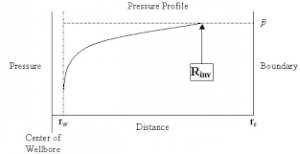The image above represents radius of investigation.

To compute for the radius of investigation, five essential parameters are needed and these parameters are permeability (k), porosity (φ), viscosity (μ), time (t) and total compressibility (CT).

The formula for calculating the radius of investigation:

rinv = 0.0325 √[Kt / φμCT]

Where:

K = Permeability
φ = Porosity
μ = Viscosity
t = Time
CT = Total Compressibility

Let’s solve an example;
Find the radius of investigation when the permeability is 25, porosity is 18, viscosity is 12, time is 22 and total compressibility is 37.

This implies that;

K = Permeability = 25
φ = Porosity =18
μ = Viscosity = 12
t = Time = 22
CT = Total Compressibility = 37

rinv = 0.0325 √[Kt / φμCT]
rinv = 0.0325 √[25 x 22 / 18 x 12 x 37]
rinv = 0.0325 √[25 x 22 / 7992]
rinv = 0.0325 √[550 / 7992]
rinv = 0.0325 √[0.068]
rinv = 0.0325 [0.26]
rinv = 0.0085

Therefore, the radius of investigation is 0.0085 ft.

Nickzom Calculator – The Calculator Encyclopedia is capable of calculating the radius of investigation.

To get the answer and workings of the radius of investigation using the Nickzom Calculator – The Calculator Encyclopedia. First, you need to obtain the app.

You can get this app via any of these means:

To get access to the professional version via web, you need to register and subscribe for NGN 1,500 per annum to have utter access to all functionalities.
You can also try the demo version via https://www.nickzom.org/calculator

Apple (Paid) – https://itunes.apple.com/us/app/nickzom-calculator/id1331162702?mt=8
Once, you have obtained the calculator encyclopedia app, proceed to the Calculator Map, then click on Petroleum under EngineeringNow, Click on Well Testing under Petroleum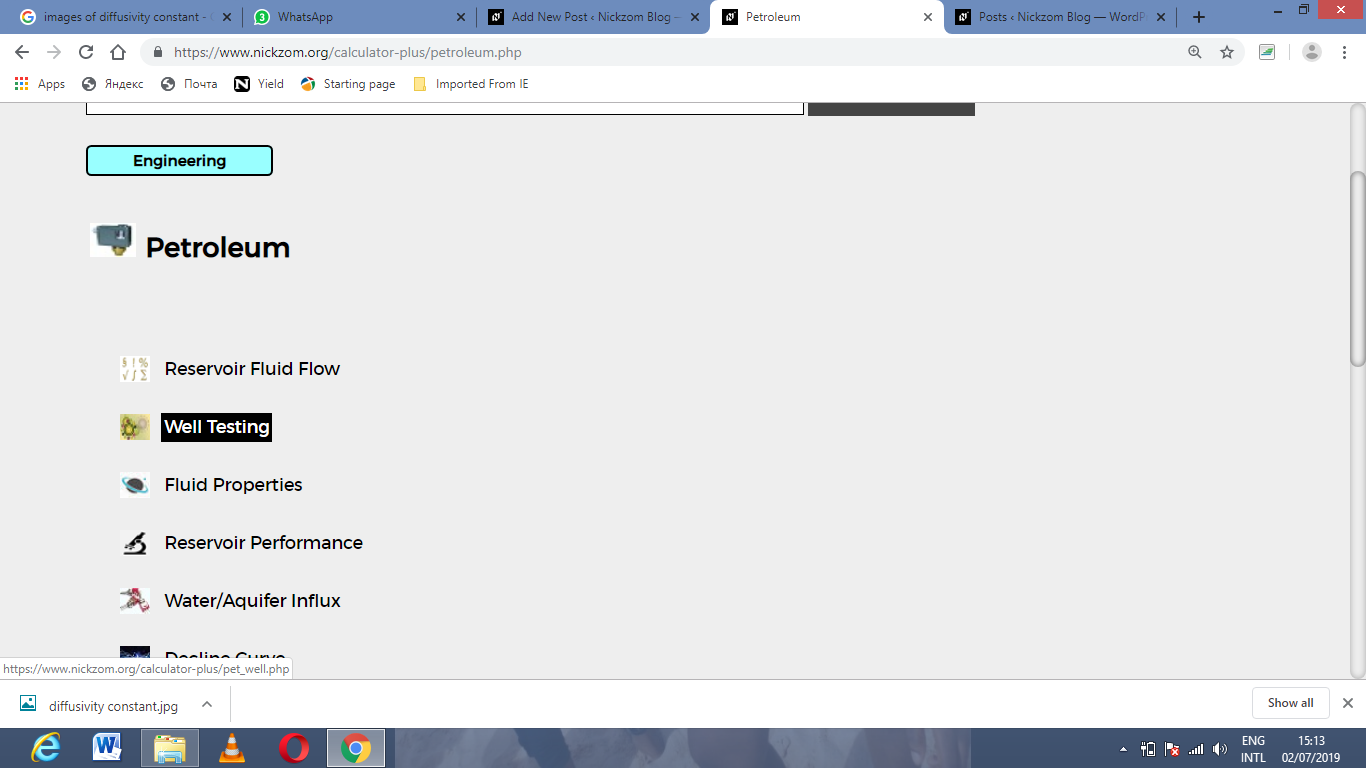Now, Click on Radius of Investigation under Well Testing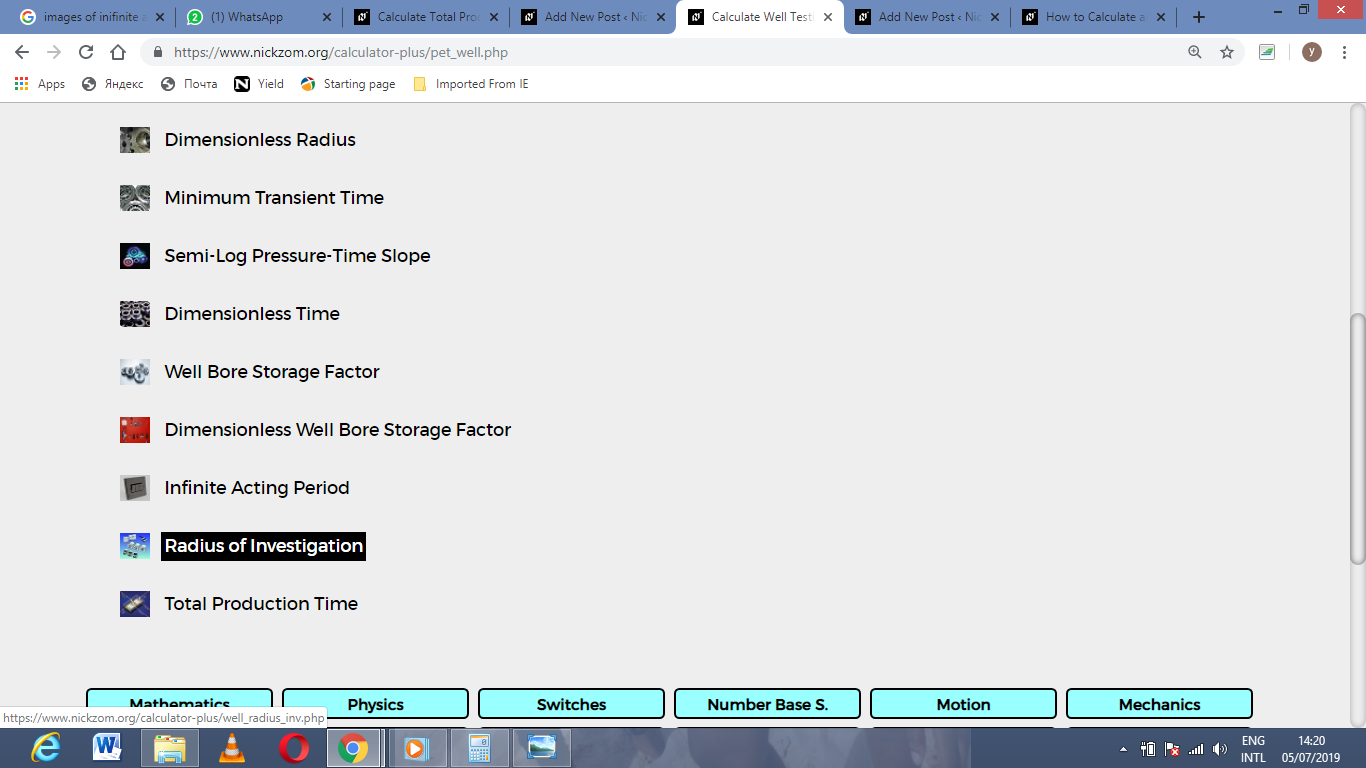The screenshot below displays the page or activity to enter your value, to get the answer for the radius of investigation according to the respective parameter which are the permeability (k), porosity (φ), viscosity (μ), time (t) and total compressibility (CT).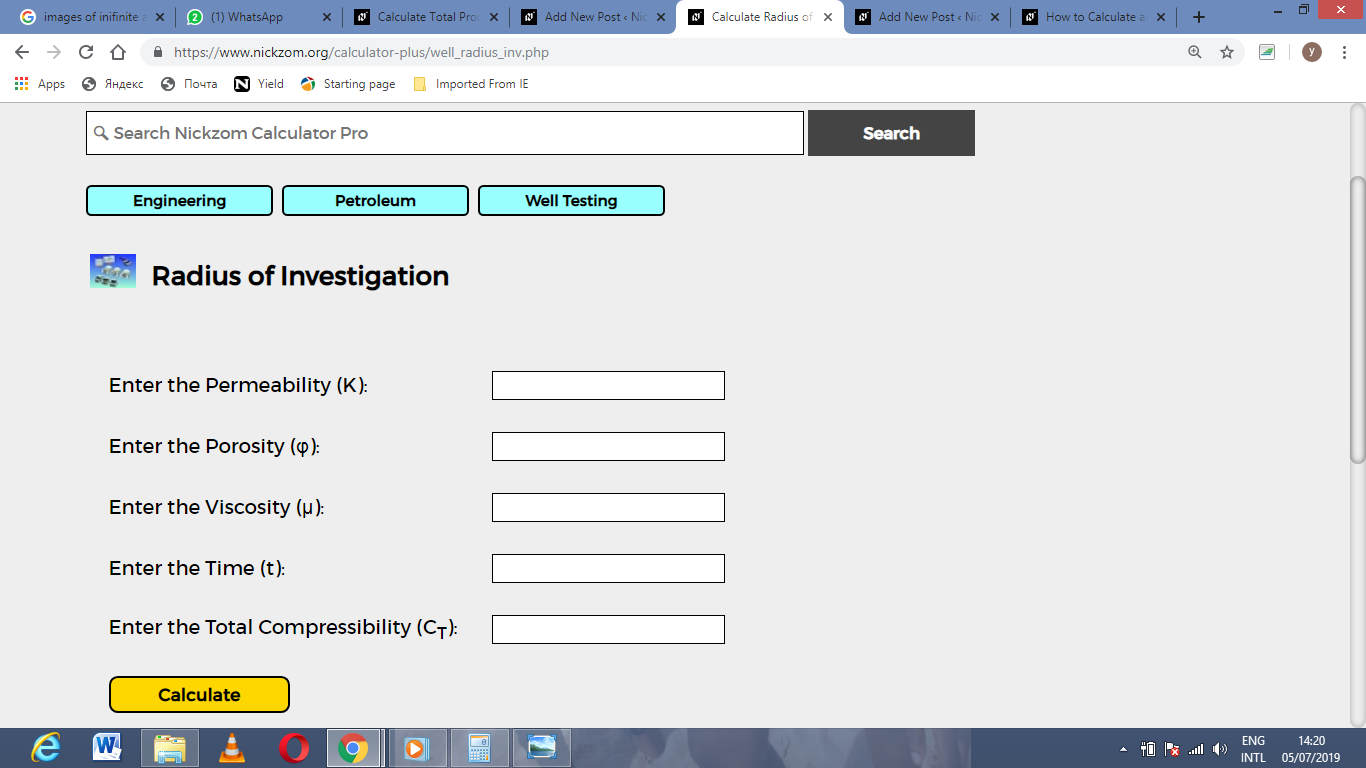Now, enter the value appropriately and accordingly for the parameter as required by the permeability (k) is 25, porosity (φ) is 18, viscosity (μ) is 12, time (t) is 22 and total compressibility (CT) is 37.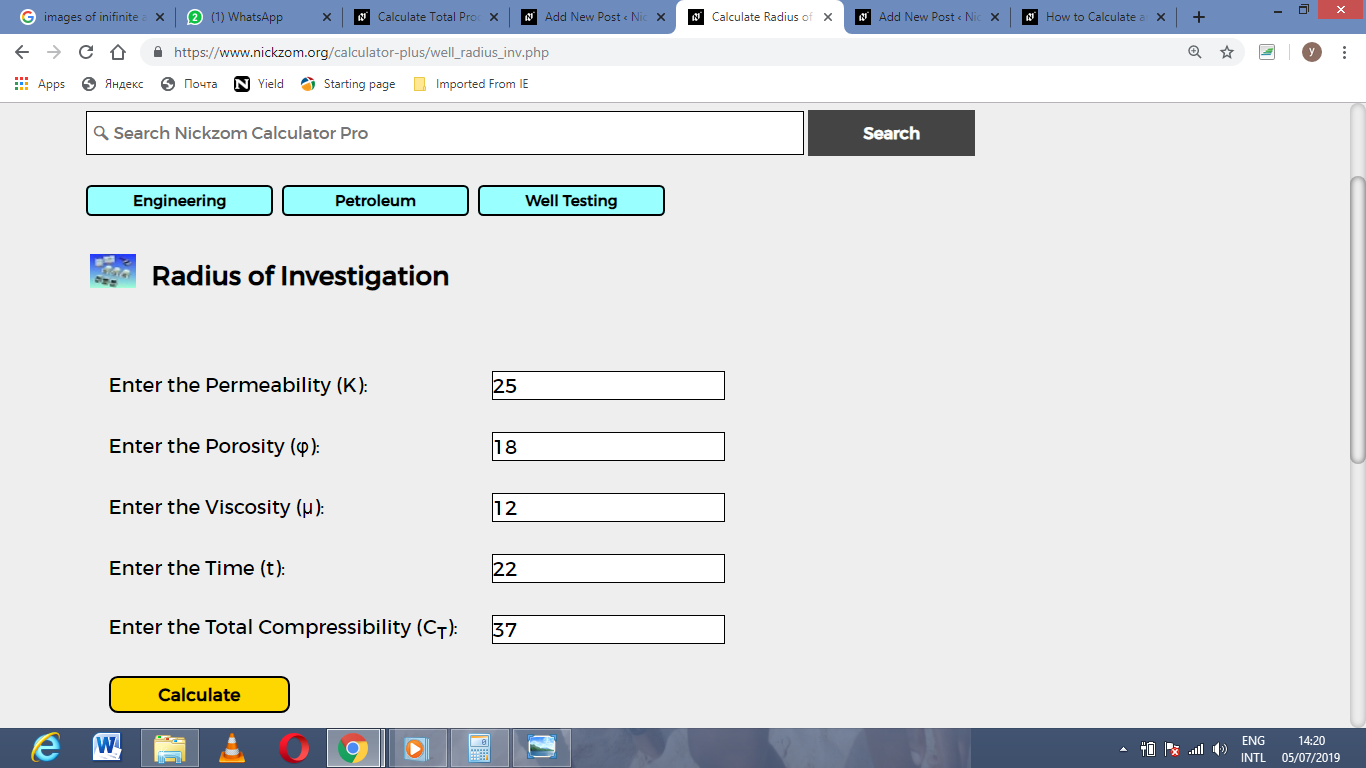Finally, Click on Calculate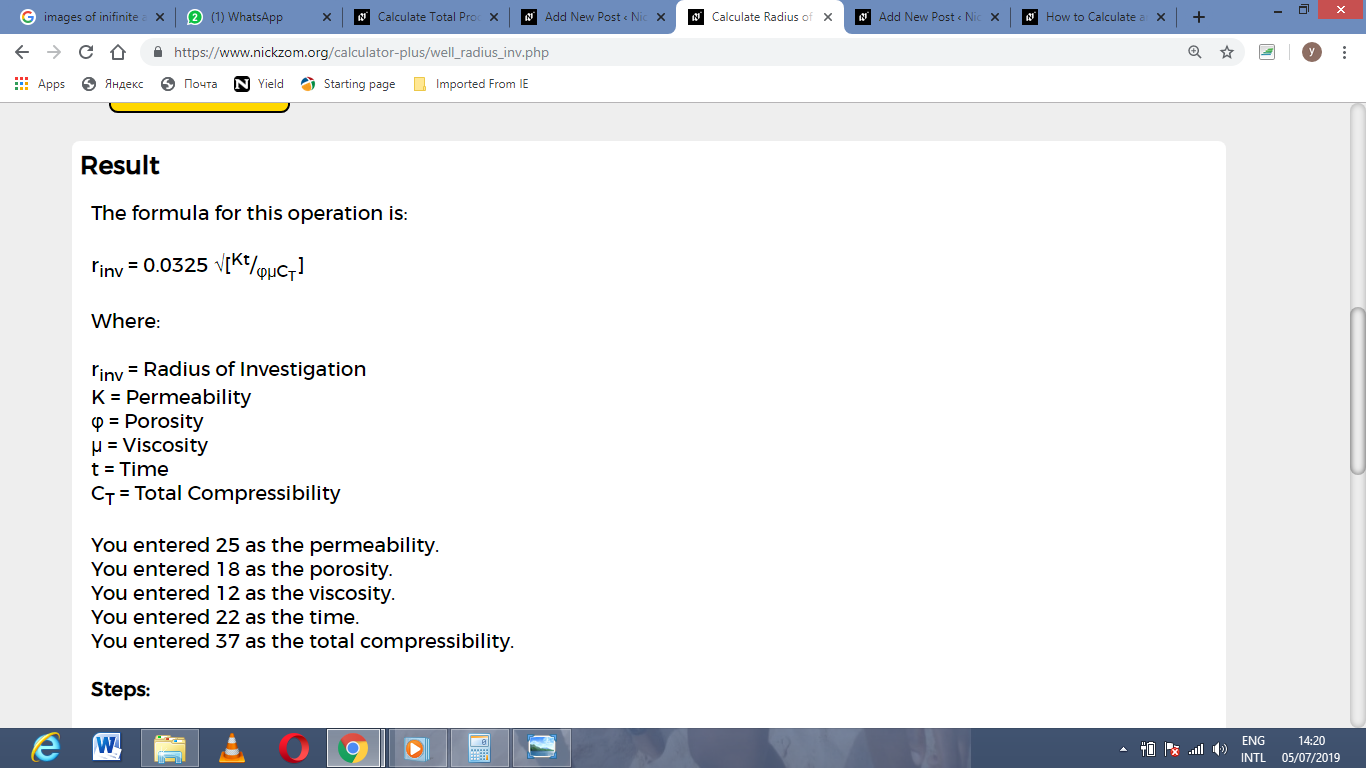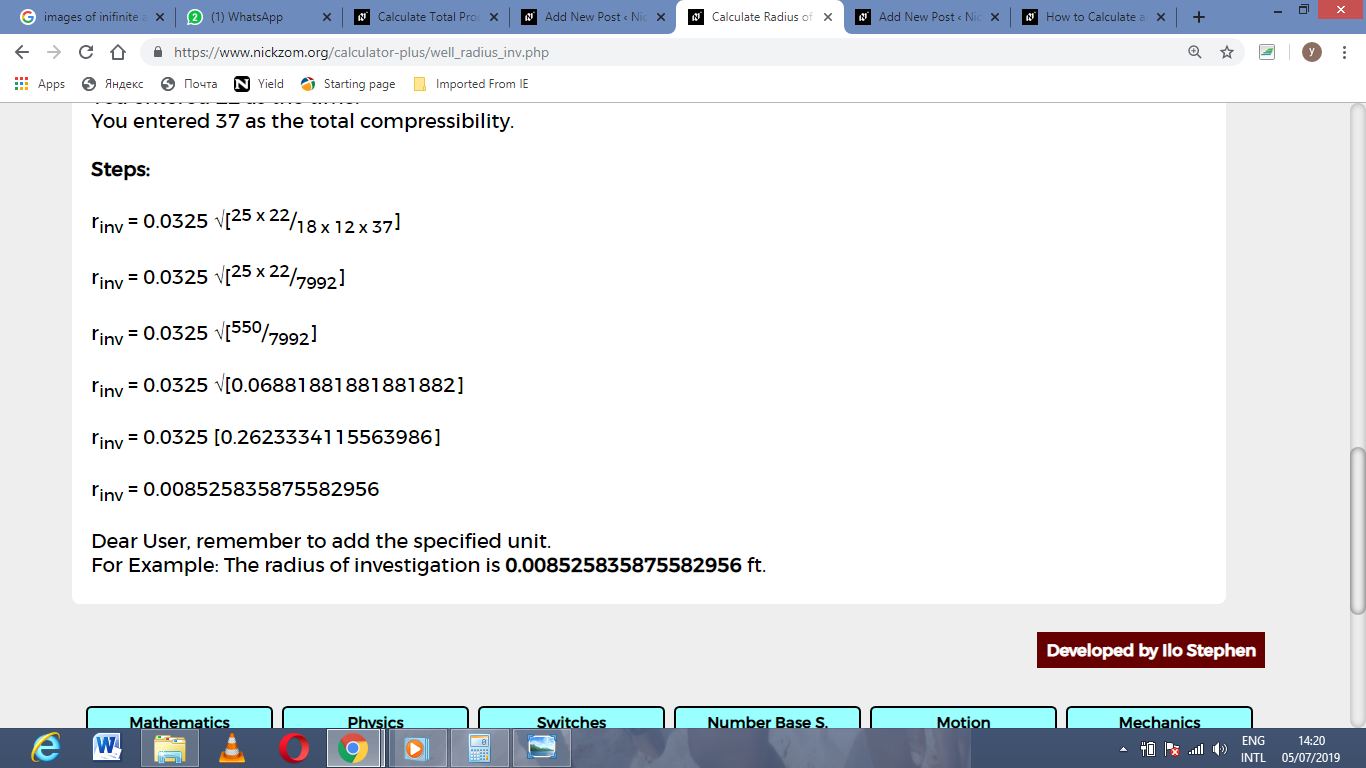As you can see from the screenshot above, Nickzom Calculator– The Calculator Encyclopedia solves for the radius of investigation and presents the formula, workings and steps too.